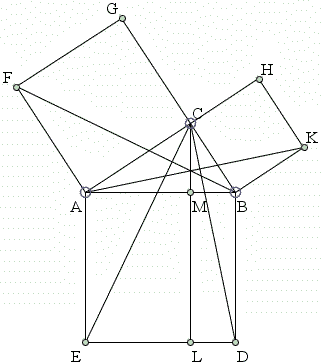## Properties of the Figures in Euclid I.47

The basic diagram that accompanies Euclid I.47 held quite naturally a lasting interest over the centuries.Some properties of the diagram and its generalizations have been already described, see Bride's Chair. Here I'll list a few more properties that came to my attention more recently.

1. Let U denote the intersection of AK and CD and V the intersection of CE and BF. Let C(ABDE) be the circle circumscribed around square ABDE. Then both U and V lie on that circle. (Solution is on a separate page.)

2. AC² + CD² = BC² + CE².

This follows from the previous property an the Law of Cosines. Let U be the intersection of AK and CD and V the intersection of CE and BF. AK⊥CD and CE⊥BF. From the Law of Cosines in triangles ACD and BCE, we have:

 AD² = AC² + CD² - 2 CV·CD BE² = BC² + CE² - 2 CU·CE

Since AD = BE, to prove the property, we only need to show that CV·CD = CU·CE. But, by the previous property, points U and V lie on the circumcircle of the square ABDE. CV·CD = CU·CE then follows by the Intersecting Secants theorem.

3. DK² = AC² + 4 BC².

Apply the Law of Cosines in ΔCDK and also in ΔACD:

 DK² = CK² + CD² - 2 CV·CD AD² = AC² + CD² - 2 CV·CD.

Subtracting the second from the first gives

 DK² = CK² + AD² - AC² = 2BC² + 2AB² - AC² = 2BC² + 2(AC² + BC²) - AC² = AC² + 4 BC².
4. DK² + EF² = 5 AB².

Assuming DK² = AC² + 4 BC², by symmetry, we then also have EF² = BC² + 4 AC². In view of the Pythagorean theorem, adding the two gives the required result.

5. Four times the sum of the squares of the medians from A and B equals 5AB².

We know that the lengths of ma, the median from A, and mb, the median from B, can be found from

 ma² = (b² + c²)/2 - a²/4, mb² = (a² + c²)/2 - b²/4.

Adding the two gives

 ma² + mb² = c² + (a² + b²) / 4 = c² · 5 / 4.
6. Let AK and BC cross in X, BF and AC cross in Y. Then CX = CY and, in addition, if CXZY is a square, then Z lies on AB.

The proof appears on a separate page.

7. DK² + GH² + EF² = 3 (AB² + BC² + AC²) [Exercices, p. 736].

This is a direct consequence of DK² + EF² = 5 AB² and GH = AB.

8. Area(EDKHGF) = 2(AC² + AC·BC + BC²).

All four triangles ABC, BDK, CGH, AFE have the same area AC·BC/2. The claim follows immediately from the Pythagorean theorem.

### References

1. F. G.-M., Exercices de Géométrie, Éditions Jacques Gabay, sixiéme édition, 1991
2. H. S. Hall, F. H. Stevens, A text-book of Euclid's elements for the use of schools, MacMillan & Co, 1904Copyright © 1996-2018 Alexander Bogomolny

 65270757